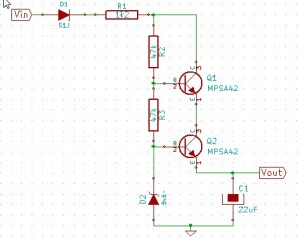## Zener diodes

Zener diodes are the oft forgotten solution to simple voltage regulation requirements.
For low current use circuits a simple Zener diode is sufficient as a voltage regulator. What’s more with the addition of a couple of transistors this “regulator” can serve as a very wide input voltage regulator where isolation isn’t required.The circuit diagram given here fills such a purpose. So let’s examine how it works.

Diode D1 is a reverse polarity protection diode which allows the circuit to be connected to both AC and DC inputs.
R1, R2 and R3 serve to bias the transistors and the Zener diode D2. R1 also limits the current into the circuit so it’s value is quite critical and depends on the load current flowing out of Vout.
The zener diode D2 determines the output voltage. Note that because the diode is connected to the output via a base-emitter junction for 5V output the zener value needs to be 5.6V.

That’s all fine but how do I derive all the component values?

First establish what the output voltage and current are to be. Say in our example 5V and 10mA respectively.
Also determine what the input voltage is to be say 24Vdc ± 20% to 240Vac ±10%.

From the output voltage we know that the zener voltage needs to be Vout+0.6 = 5.6V. That was easy

From the output current and minimum input voltage we can determine what the maximum value for R1 is to be:
R1 = (Vin_min-Vout)/Iout = (24*0.8 – 5)/0.01 = 1420Ω take the nearest lower standard value that you may have say 1k2.

R2 and R3 aren’t critical as such and 47kΩ is a good value for biasing the zener diode as well as the transistors. Basically you want to have a little current flowing through them as possible yet still guarantee that the zener is operating at its nominal voltage.

Q1 and Q2 need to be high voltage bipolar transistors if the circuit is to be connected to mains (300V rating). The same goes for the resistors and D1.

C1 filters out the ripple when the circuit is supplied by AC and depends on the output current and acceptable ripple voltage.

A note of caution though: although the current flowing through R1, R2 and R3 is only small (~10mA) they dissipate power. In our example R1 will dissipate roughly 1200*0.01²=0.12W So to be on the safe side and avoid resistors burning use ½W rated ones.

Finally a note on safety: when connecting this circuit to mains all the parts are referenced with respect of neutral and thus is dangerous. Do not touch any parts of the circuit when energised.